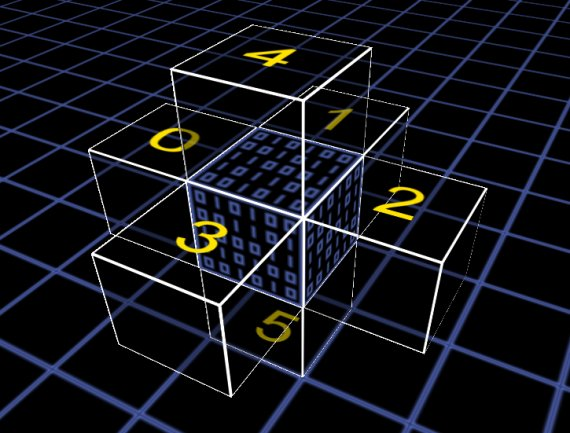1. # Move

## Description

integer Move(integer Location)

Instructs the robot to move of one voxel. You can move the robot only once per cycle (per Voxel_Step() call). You can move only to non solid locations. This function can only be used in Voxel_Step().

## Parameters

Location : The position where to move in "position code" notation.

0 =  { 0, 0, 1 } = Front of the robot.
1 =  { 1, 0, 0 } = Right of the robot.
2 =  { 0, 0, -1} = Back of the robot.
3 =  {-1, 0, 0 } = Left of the robot.
4 =  { 0, 1, 0 } = Above the robot.
5 =  { 0,-1, 0 } = Under the robot.## Return Value

A boolean wich is true if the robot moved successfully or false otherwise.

## Example of use

```// Listing #1: Random Mover Robot
function Voxel_Step()
{
Move( GetRnd(3) );
}

```